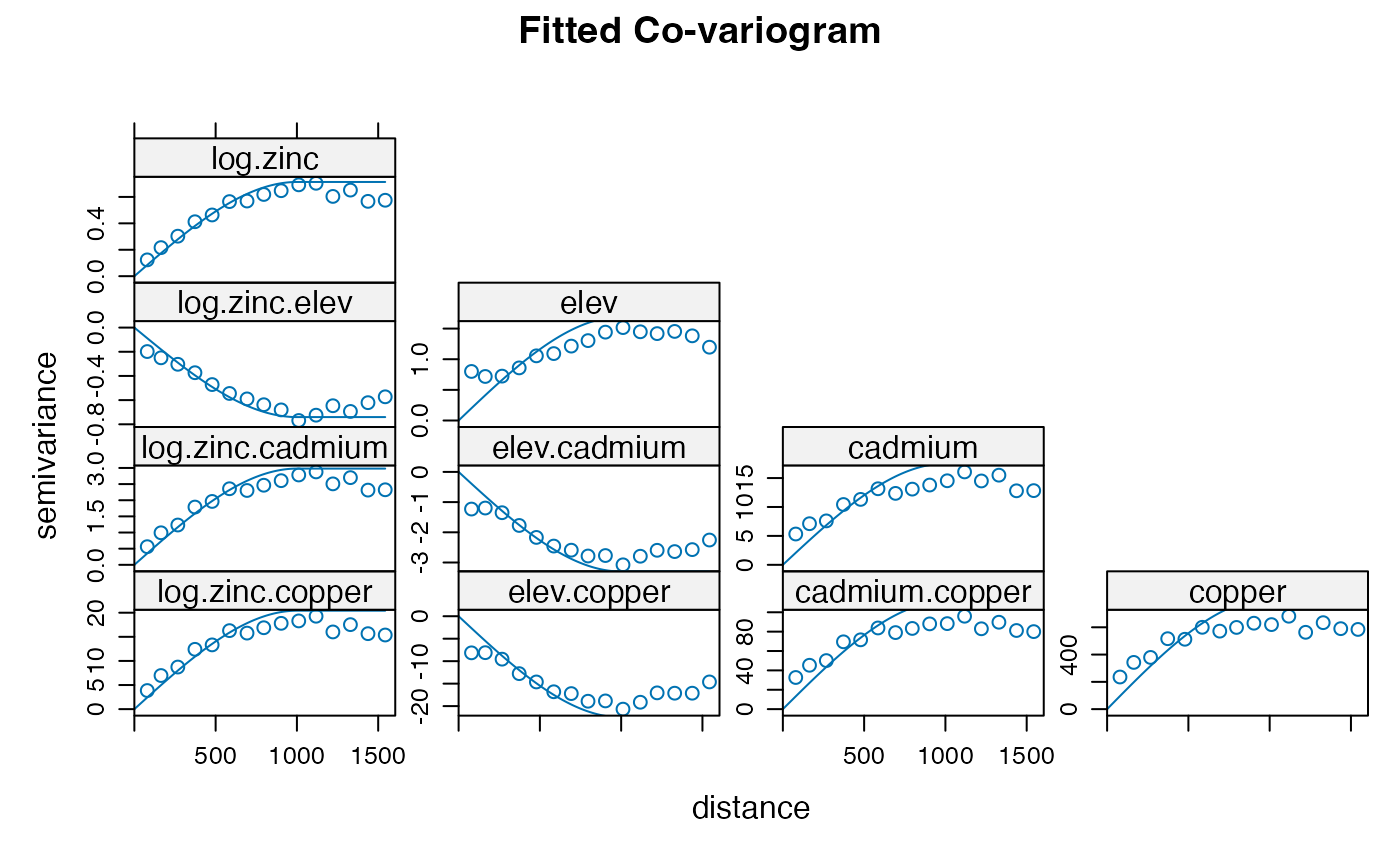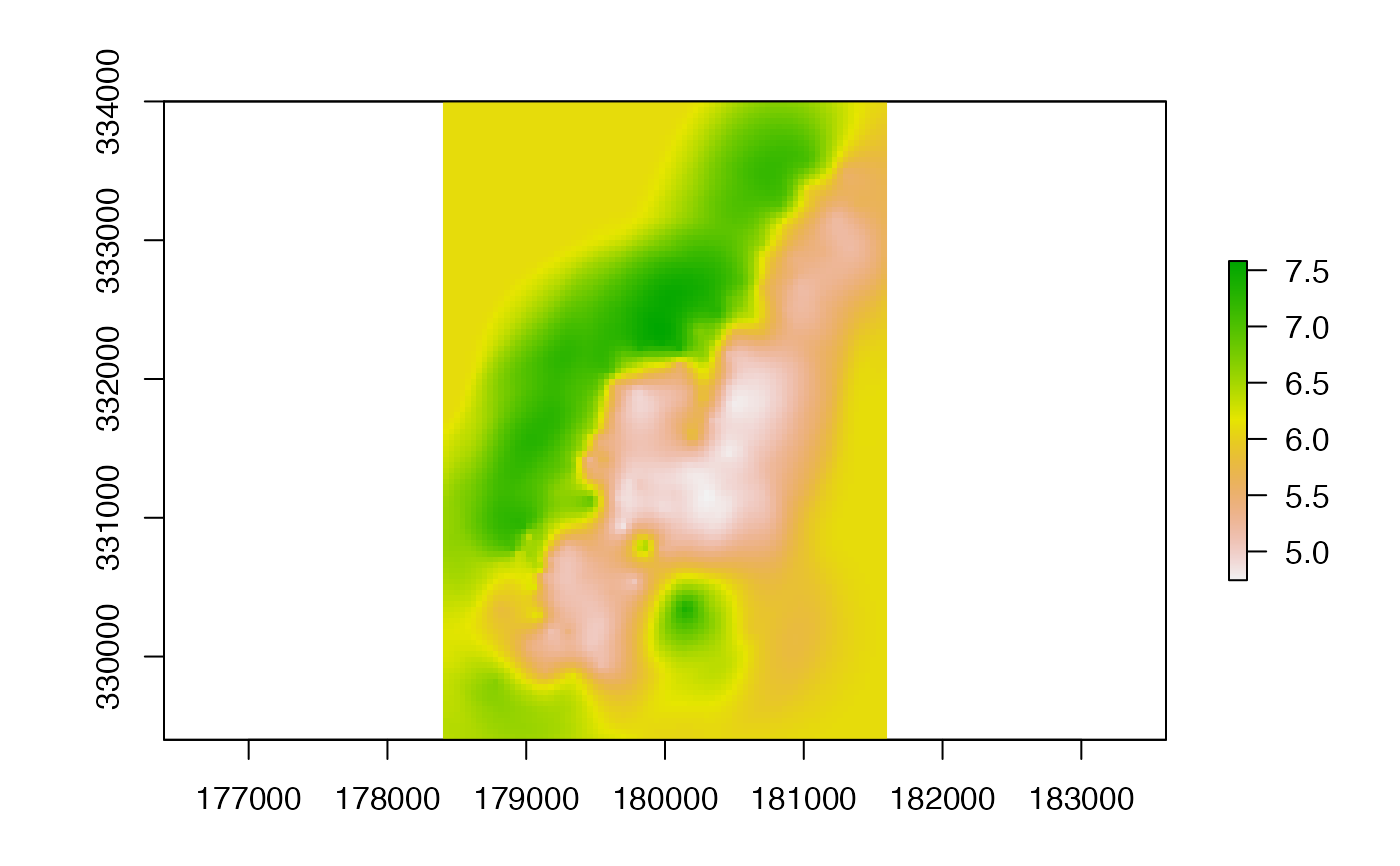Make a RasterLayer with interpolated values using a fitted model object of classes such as 'gstat' (gstat package) or 'Krige' (fields package). That is, these are models that have location ('x' and 'y', or 'longitude' and 'latitude') as independent variables. If x and y are the only independent variables provide an empty (no associated data in memory or on file) RasterLayer for which you want predictions. If there are more spatial predictor variables provide these as a Raster* object in the first argument of the function. If you do not have x and y locations as implicit predictors in your model you should use predict instead.

# S4 method for Raster
interpolate(object, model, filename="", fun=predict, xyOnly=TRUE,
xyNames=c('x', 'y'), ext=NULL, const=NULL, index=1, na.rm=TRUE, debug.level=1, ...)

## Arguments

object

Raster* object

model

model object

filename

character. Output filename (optional)

fun

function. Default value is 'predict', but can be replaced with e.g. 'predict.se' (depending on the class of the model object)

xyOnly

logical. If TRUE, values of the Raster* object are not considered as co-variables; and only x and y (longitude and latitude) are used. This should match the model

xyNames

character. variable names that the model uses for the spatial coordinates. E.g., c('longitude', 'latitude')

ext

Extent object to limit the prediction to a sub-region of x

const

data.frame. Can be used to add a constant for which there is no Raster object for model predictions. This is particulary useful if the constant is a character-like factor value

index

integer. To select the column if 'predict.model' returns a matrix with multiple columns

na.rm

logical. Remove cells with NA values in the predictors before solving the model (and return NA for those cells). In most cases this will not affect the output. This option prevents errors with models that cannot handle NA values

debug.level

for gstat models only. See ?

...

additional arguments passed to the predict.'model' function

## Value

Raster* object

predict, predict.gstat, Tps

## Examples

# \donttest{
## Thin plate spline interpolation with x and y only
# some example data
r <- raster(system.file("external/test.grd", package="raster"))
ra <- aggregate(r, 10)
xy <- data.frame(xyFromCell(ra, 1:ncell(ra)))
v <- getValues(ra)
# remove NAs
i <- !is.na(v)
xy <- xy[i,]
v <- v[i]

#### Thin plate spline model
library(fields)
#> Spam version 2.10-0 (2023-10-23) is loaded.
#> Type 'help( Spam)' or 'demo( spam)' for a short introduction
#> and overview of this package.
#> Help for individual functions is also obtained by adding the
#> suffix '.spam' to the function name, e.g. 'help( chol.spam)'.
#>
#> Attaching package: ‘spam’
#> The following objects are masked from ‘package:base’:
#>
#>     backsolve, forwardsolve
#>
#> Try help(fields) to get started.
tps <- Tps(xy, v)
p <- raster(r)

# use model to predict values at all locations
p <- interpolate(p, tps)

plot(p)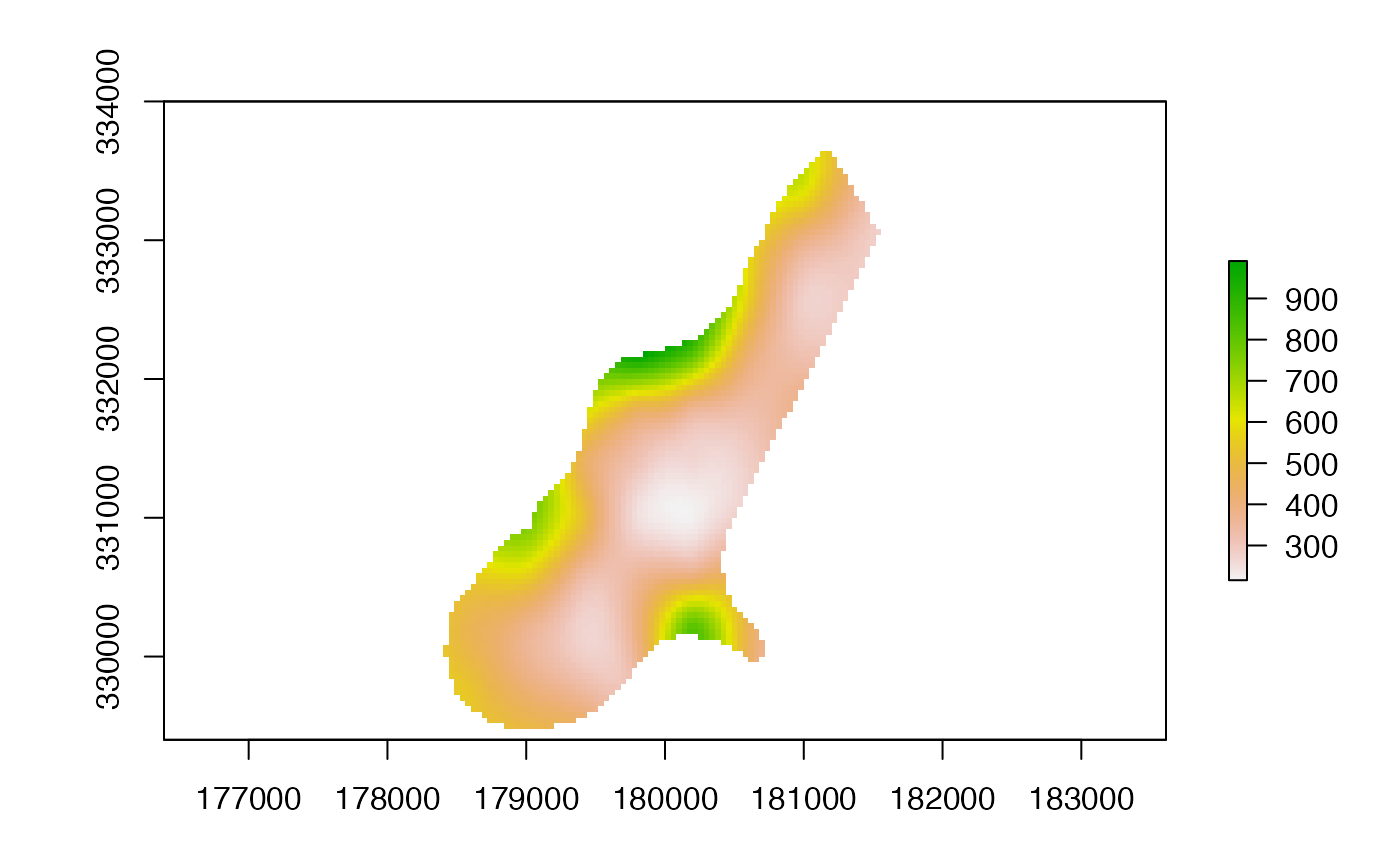## change the fun from predict to fields::predictSE to get the TPS standard error
se <- interpolate(p, tps, fun=predictSE)
plot(se)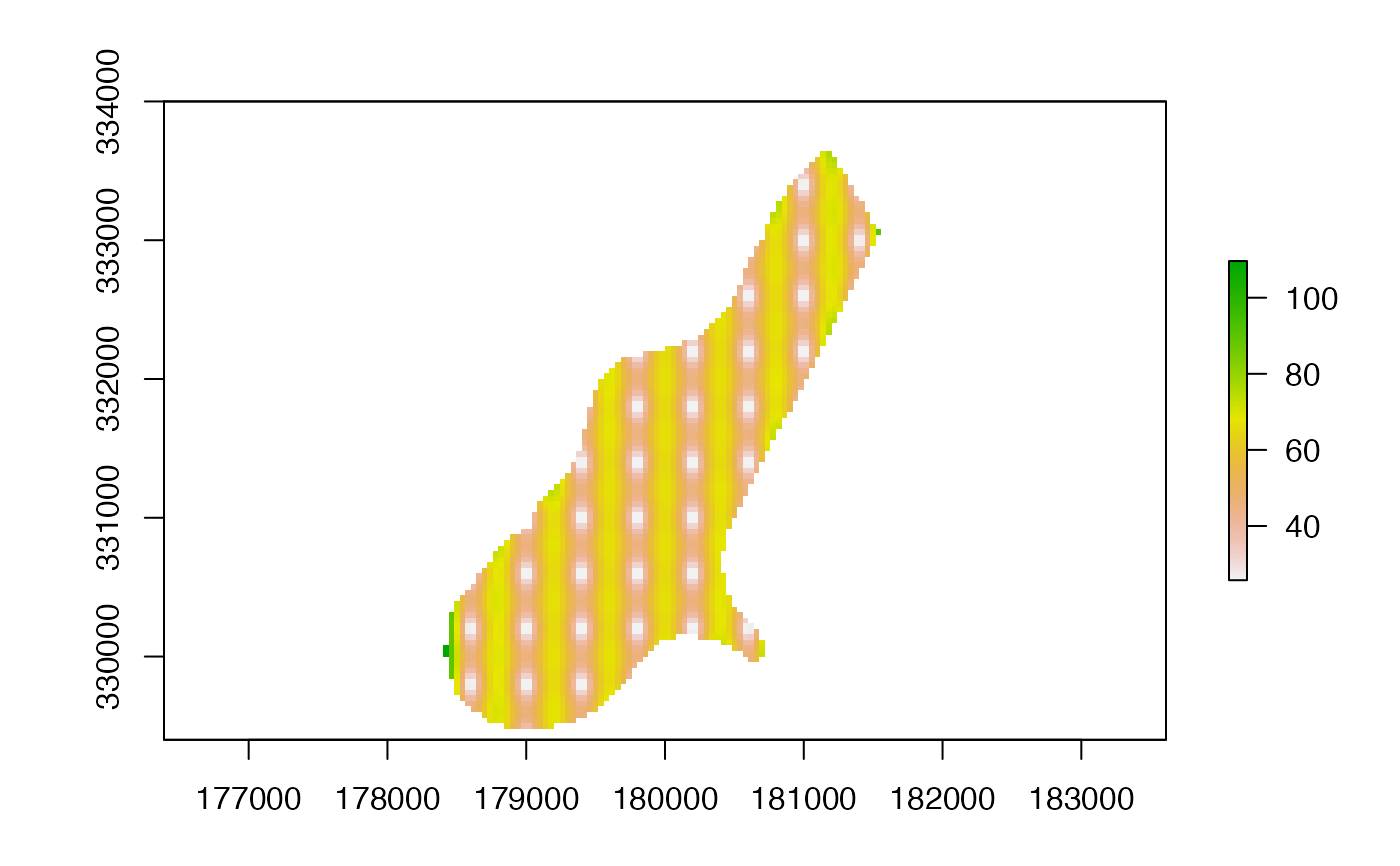## another variable; let's call it elevation
elevation <- (init(r, 'x') * init(r, 'y')) / 100000000
names(elevation) <- 'elev'

z <- extract(elevation, xy)

# add as another independent variable
xyz <- cbind(xy, z)
tps2 <- Tps(xyz, v)
p2 <- interpolate(elevation, tps2, xyOnly=FALSE)

# as a linear coveriate
tps3 <- Tps(xy, v, Z=z)

# Z is a separate argument in Krig.predict, so we need a new function
# Internally (in interpolate) a matrix is formed of x, y, and elev (Z)

pfun <- function(model, x, ...) {
predict(model, x[,1:2], Z=x[,3], ...)
}
p3 <- interpolate(elevation, tps3, xyOnly=FALSE, fun=pfun)

#### gstat examples
library(gstat)
data(meuse)

## inverse distance weighted (IDW)
r <- raster(system.file("external/test.grd", package="raster"))
data(meuse)
mg <- gstat(id = "zinc", formula = zinc~1, locations = ~x+y, data=meuse,
nmax=7, set=list(idp = .5))
z <- interpolate(r, mg)
#> [inverse distance weighted interpolation]

## kriging
coordinates(meuse) <- ~x+y
crs(meuse) <- crs(r)

## ordinary kriging
v <- variogram(log(zinc)~1, meuse)
m <- fit.variogram(v, vgm(1, "Sph", 300, 1))
gOK <- gstat(NULL, "log.zinc", log(zinc)~1, meuse, model=m)
OK <- interpolate(r, gOK)
#> [using ordinary kriging]

# examples below provided by Maurizio Marchi
## universial kriging
vu <- variogram(log(zinc)~elev, meuse)
mu <- fit.variogram(vu, vgm(1, "Sph", 300, 1))
gUK <- gstat(NULL, "log.zinc", log(zinc)~elev, meuse, model=mu)
names(r) <- 'elev'
UK <- interpolate(r, gUK, xyOnly=FALSE)
#> [using universal kriging]

## co-kriging
gCoK <- gstat(NULL, 'log.zinc', log(zinc)~1, meuse)
gCoK <- gstat(gCoK, 'elev', elev~1, meuse)
gCoK <- gstat(gCoK, 'copper', copper~1, meuse)
coV <- variogram(gCoK)
plot(coV, type='b', main='Co-variogram')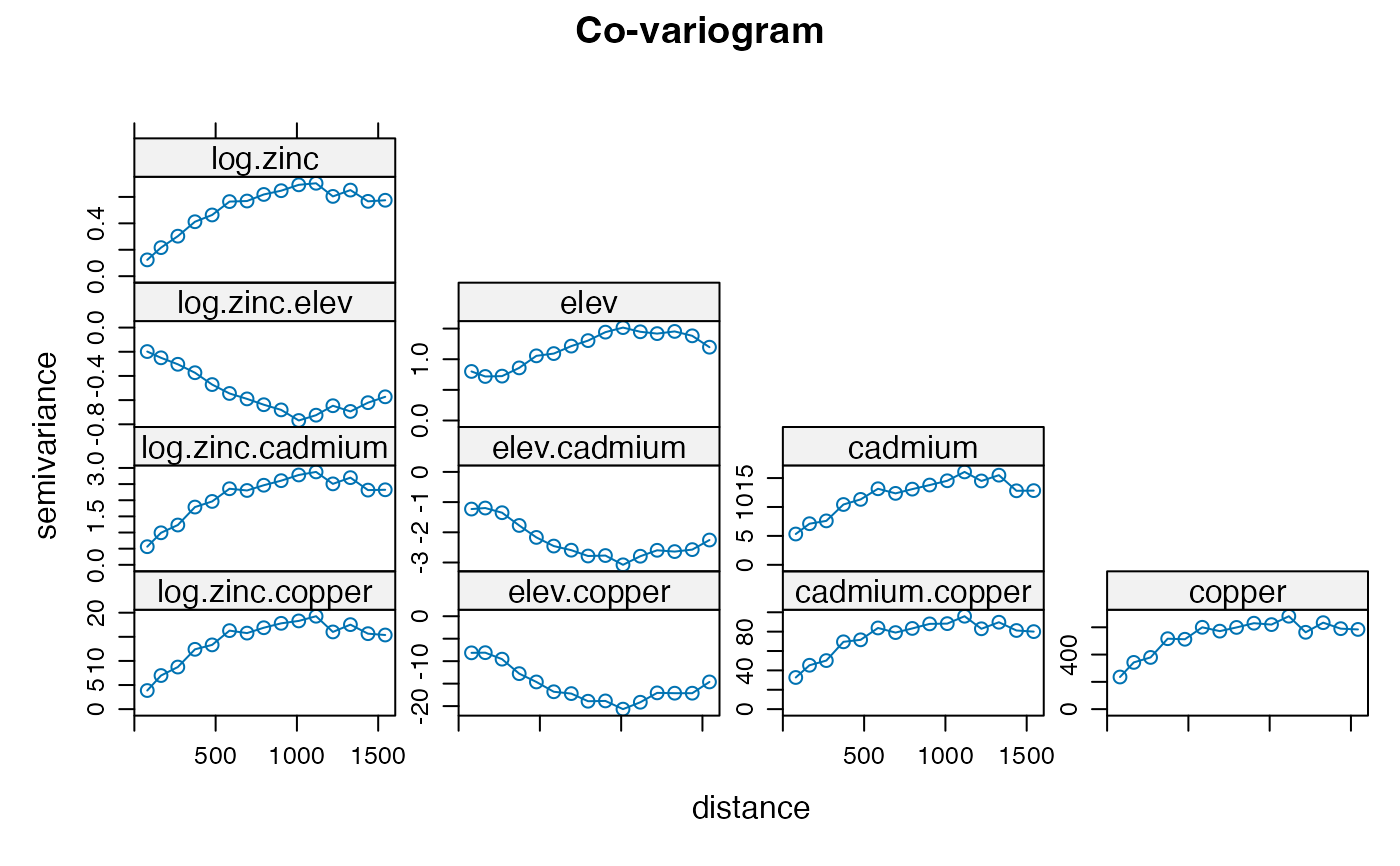coV.fit <- fit.lmc(coV, gCoK, vgm(model='Sph', range=1000))
coV.fit
#> data:
#> log.zinc : formula = log(zinc)~1 ; data dim = 155 x 12
#> elev : formula = elev~1 ; data dim = 155 x 12
#> cadmium : formula = cadmium~1 ; data dim = 155 x 12
#> copper : formula = copper~1 ; data dim = 155 x 12
#> variograms:
#>                  model       psill range
#> log.zinc           Sph   0.7132435  1000
#> elev               Sph   1.6908552  1000
#> copper             Sph 809.4027563  1000
#> log.zinc.elev      Sph  -0.7404289  1000